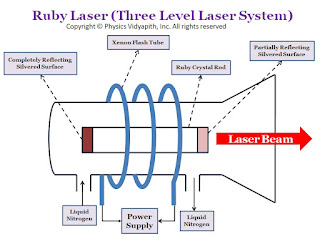Showing posts with label Magnetism. Show all posts
Showing posts with label Magnetism. Show all posts

## Magnetic Field at the axis of a current-carrying Circular Loop

Derivation→

Let us consider, A circular loop which have radius $a$, carrying a current $i$. Let take a point $P$ on the axis of the loop at a distance $x$ from the center $O$ of the loop.

Now to find the magnetic field at point $P$, take a small current element of length $dl$ at the top of the loop. Let $r$ is the distance between the current element and point $P$. Now apply the Biot-Savart's Law to find the magnitude of the magnetic field due to small current-element $dl$ at point $P$ i.e.

$dB=\frac{\mu_{\circ}}{4 \pi} \frac{i \: dl\: sin\theta}{r^{2 }}$

Where $\theta$ is the angle between the length of the element $dl$ and the line joining the element to the point $P$. Here this angle is $90^{\circ}$

$dB=\frac{\mu_{\circ}}{4\pi} \frac{i \: dl}{r^{2}}\qquad(1)$

The direction of the magnetic field $d\overrightarrow{B}$ is in the plane of the paper and at right angles to the line $r$ as shown in the figure below (By applying the right-hand screw rule). It can be resolved into two components, one of magnitude $dB\: sin\phi$ along the axis of the loop and the other of magnitude $dB\: cos\phi$ at right angles to the axis.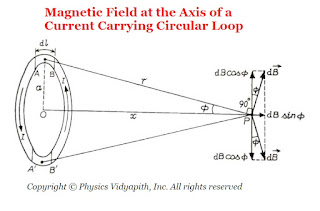Magnetic Field at the Axis of a Current-Carrying Circular Loop

Let us consider another current element of the same length at the bottom of the loop, directly opposite to the first element, which is at right angles to the plane of the page but directed inwards. the field due to $d\overrightarrow{B}$ but directed as shown in the figure above. It is clear that components of both the fields at right angles to the axis are equal and opposite. Hence they cancel each other. The component along the axis are in the same direction and so they are added up.

If we imagine the whole of the loop to be divided into such current elements, the resultant field $\overrightarrow{B}$ at $P$ is directed along the axis and its magnitude is given by

$B=\int dB \: \sin\phi$

Now substitute the value of $dB$ from equation $(1)$

$B=\int \frac{\mu_{\circ}}{4\pi} \frac{i \: dl}{r^{2}} \: \sin\phi$

From figure, $sin\phi=\frac{a}{r}$, we get

$dB=\int \frac{\mu_{\circ}}{4 \pi} \frac{i}{r^{2}} \frac{a}{r}dl$

$dB=\int \frac{\mu_{\circ}}{4 \pi} \frac{ia}{r^{3}} dl$

$dB= \frac{\mu_{\circ}}{4 \pi} \frac{ia}{r^{3}} \int dl$

$dB= \frac{\mu_{\circ}}{4 \pi} \frac{ia}{r^{3}} 2 \pi \: a \qquad \left( \int dl = 2 \pi \: a \right)$

$dB= \frac{\mu_{\circ}ia^{2}}{2r^{3}}$

Agian from figure $r^{2}=\left( a^{2} + x^{2}\right)^{3}$ or $r= \left( a^{2} + x^{2}\right)^{3/2}$. Now substitute the value of $r$ in above equation.

 $B=\frac{\mu_{\circ}\: i\: a^{2}}{2(a^{2}+x^{2})^{3/2}}$

If it is a coil of $N$ turns, then each turn will contribute equally to $B$. Thus

 $B=\frac{\mu_{\circ}\: N \: i \: a^{2}}{2(a^{2}+x^{2})^{3/2}}$

The direction of the magnetic field $\overrightarrow{B}$ is along the axis of the loop and that of the coil.

Magnetic field at the Centre of the Loop→

At the centre of the coil, we have $x=0$

 $B=\frac{\mu_{\circ}\: N \: i}{2a}$

Again the direction of the magnetic field $\overrightarrow{B}$ is perpendicular to the plane of the coil i.e. Along the axis of the coil.

## Magnetic Field due to a Straight Current-Carrying Conductor of Finite Length

Derivation→

Let us consider, a current-carrying conductor $XY$ having length $l$ in which current $i$ is flowing from $X$ to $Y$. Now, To find the magnetic field due to the conductor, take a point $P$ at a distance $d$ from point $O$ of the conductor.

Now consider a small length element $dl$ at the conductor which is making an angle $\theta$ from point $P$. The length element $dl$ is also making the angle $d\theta$ from point $O$. If $\theta_{1}$ and $\theta_{2}$ is the angle from point $O$ to point $X$ and $Y$ respectively. Than magnetic field at point $P$ due to small length element $dl$ which is at a distance $r$

$dB=\frac{\mu_{0}}{4\pi} \frac{i\: dl \: sin (90+\theta)}{r^{2}}$

$dB=\frac{\mu_{0}}{4\pi} \frac{i \: dl \: cos\theta}{r^{2}} \qquad(1)$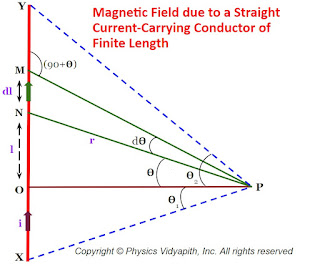Magnetic Field due to a Straight Current-Carrying Conductor of Finite Length

But from the figure, In $\Delta NOP$

$cos\theta=\frac{d}{r}$

$r=\frac{d}{cos\theta} \qquad(2)$

$tan\theta=\frac{l}{d}$

$l=d\:tan\theta$

Now differentiate the above equation with respect to $\theta$, then

$dl=d \: sec^{2}\theta \: d\theta \qquad(3)$

Now substitute the value of $dl$ and $r$ from equation$(2)$ and equation $(3)$ in equation$(1)$, then we get

$dB=\frac{\mu_{o}i}{4\pi}\frac{d \:sec^{2}\theta \: d\theta \: cos\theta}{\frac{d^{2}}{cos^{2}\theta}}$

$dB=\frac{\mu_{o}i}{4\pi}\frac{sec^{2}\theta \: d\theta \: cos^{3}\theta}{d}$

$dB=\frac{\mu_{o}i}{4\pi}\frac{cos\theta \: d\theta}{d} \qquad(4)$

The magnitude of the magnetic field $\overrightarrow{B}$ at point $P$ due to the whole conductor $XY$ is

$B=\int_{-\theta_{1}}^{\theta_{2}}dB$

Now substitute the value of $dB$ in above equation so

$B=\int_{-\theta_{1}}^{\theta_{2}} \frac{\mu_{o}i}{4\pi}\frac{cos\theta \: d\theta}{d}$

$B=\int_{-\theta_{1}}^{\theta_{2}} \frac{\mu_{o}i}{4\pi d}cos\theta d\theta$

$B= \frac{\mu_{o}i}{4\pi d} \left[ sin\theta\right] _{-\theta_{1}} ^{\theta_{2}}$

$B= \frac{\mu_{o}i}{4\pi d} \left[ sin(\theta _{2}) - sin(-\theta_{1})\right]$

 $B=\frac{\mu_{o}i}{4\pi d}\left( sin\theta _{1} + sin\theta_{2}\right)$

Special Case→

1. For a conductor of infinite length, i.e $\theta_{1}=\theta_{2}=90^{\circ}$ then magnetic field

$B=\frac{\mu_{0}}{4\pi} \frac{2i}{d}$

 $B=\frac{\mu_{0}}{2\pi} \frac{i}{d}$

2. If the point $P$ is at a distance $d$ near one end of the conductor,i.e. $\theta_{1}=90^{\circ}$ and $\theta_{2}=0$ then magnetic field

 $B=\frac{\mu_{0}}{4\pi} \frac{i}{d}$

From the above equations, we can conclude that the magnetic field $B$ at point $P$ is proportional to the current $i$ and inversely proportional to the distance $d$ of point $P$ from the conductor.

The variation of the magnitude of magnetic field $B$ with distance $d$ is shown in the figure below→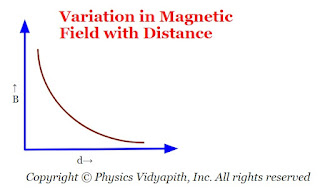Variation in Magnetic field with distance

The line of force of magnetic field $\overrightarrow{B}$ near a linear current-carrying conductor are concentric circles of varying radii with their centers at the conductor in a plane perpendicular to the conductor. The direction of the magnetic field $\overrightarrow{B}$ at a point $P$, distant $d$, will be along the tangent drawn on a circle of radius $d$ around the conductor.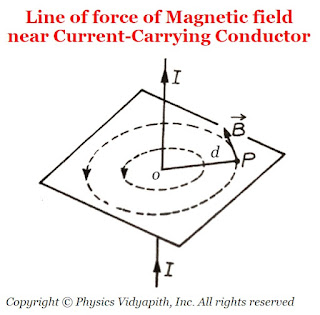Line of force of Magnetic field near Current-Carrying Conductor

## Motion of Charged Particles in Uniform Magnetic Field

A.) When $\overrightarrow{v}$ is perpendicular to $\overrightarrow{B}$→

Let us consider a charge $q$ enters in the magnetic field $\overrightarrow{B}$ from a point $O$ with velocity $\overrightarrow{v}$ directed perpendicular to the magnetic field $\overrightarrow{B}$.The general Expression for the force acting on the particle is

$\overrightarrow{F}=q(\overrightarrow{v} \times \overrightarrow{B})$

Here the magnetic field $\overrightarrow{B}$ is perpendicular to the plane of the page directed downwards which is shown in the figure below.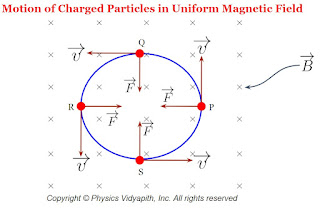The motion of Charged Particles in Uniform Magnetic Field

Since $\overrightarrow{v}$ is perpendicular to $\overrightarrow{B}$ then the magnitude of the force will be

$F=qvB \qquad(1)$

Since the force is perpendicular to the velocity and the direction of velocity continuously changes but the magnitude of velocity is not changing. Therefore the particle will move in a circular path with constant speed in a uniform magnetic field and the magnetic force will act as a centripetal force. i.e.

$F=\frac{m v^{2}}{r} \qquad(2)$

Where
$r$ → Radius of the circular path
$m$ → mass of the particle

From equation $(1)$ and equation $(2)$

$\frac{m v^{2}}{r}=qvB$

 $r=\frac{mv}{qB}$

The direction of the path of the particle depends upon the nature of the charge and the direction of the magnetic field.

The particle travels a distance $2\pi r$ in one revolution. So the time period of one revolution is

$T=\frac{2\pi r}{v} \qquad(3)$

Now substitute the value of $r=\frac{mv}{qB}$ in equation $(3)$ then we get

 $T=\frac{2\pi m}{qB}$

The frequency of the particle $n=\frac{1}{T}$ can be written as

 $n=\frac{qB}{2\pi m}$

From the above equations, We can conclude that the time period or frequency of the particle is independent of the speed $v$ of the particle. If the speed of the particle increases, its radius also increases so that the time taken to complete one revolution remains the same. If two identical charged particles enter the field with different speeds $v_{1}$ and $v_{2}$, then they move along the circle of smaller and larger radii respectively which is shown in the figure below.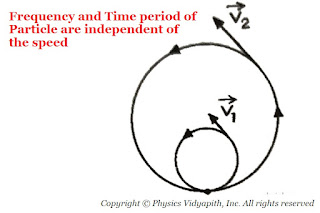The frequency and Time period of the particle are independent of the speed
B.) When $\overrightarrow{v}$ is not perpendicular to $\overrightarrow{B}$→

Now, Again Let that the particle is entering with velocity $\overrightarrow{v}$ in the magnetic field $\overrightarrow{B}$, instead of being perpendicular to $\overrightarrow{B}$, makes angle $\theta (0 < \theta < 90^{\circ})$ with it. Now the velocity $\overrightarrow{v}$ may resolved into two components;

First is:

$v_{\parallel}=v \: cos\theta$ i.e. Parallel to magnetic field $\overrightarrow{B}$. This component gives the linear path to the particle.

Second is:

$v_{\perp}=v \: sin\theta$ i.e. Perpendicular to magnetic field $\overrightarrow{B}$. This component gives the circular path to the particle.

The resultant of these two-component gives the helical path to the particle whose axis is parallel to the magnetic field.

The radius of the circular path of the helix is

$r=\frac{mv_{\perp}}{qB}$

 $r=\frac{mv \: sin\theta}{qB}$Helical Motion of Charged Particle

The time period of the particle

$T=\frac{2\pi m}{qB}$

The linear distance traveled by the particle in the direction of the magnetic field in one complete circle is called the 'pitch $(p)$' of the path.

$p=v_{\parallel} \times T$

 $T= v \: cos\theta \times \frac{2\pi m}{qB}$

Kinetic Energy of Charged Particle Moving in Uniform Magnetic Field→

The kinetic energy is

$K=\frac{1}{2}mv^{2}$

$K=\frac{(mv)^{2}}{2m}$

Now substitute the value of $mv$ from $r=\frac{mv}{qB}$. Then we get

$K=\frac{1}{2}m \left( \frac{rqB}{m} \right)^{2}$

 $K=\frac{r^{2}q^{2}B^{2}}{2m}$

### Principle, Construction and Working of the Ruby Laser